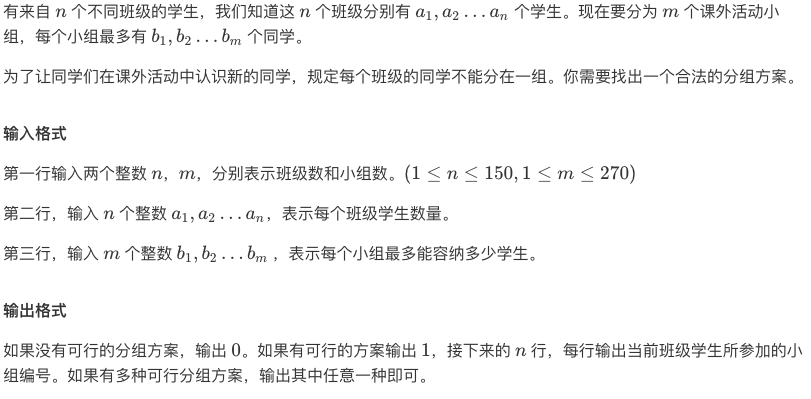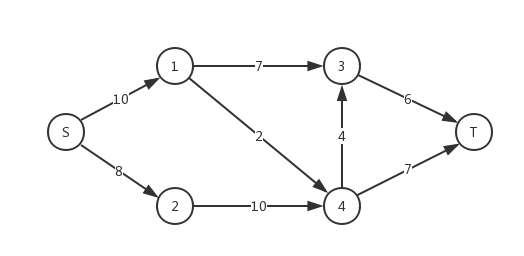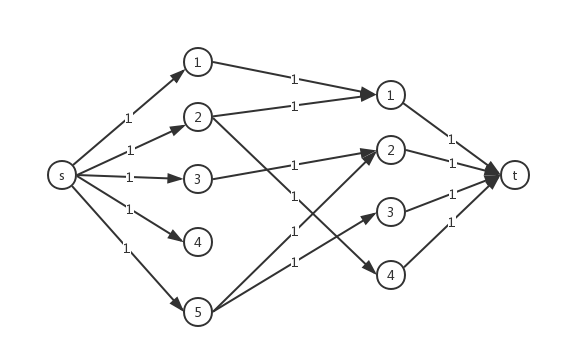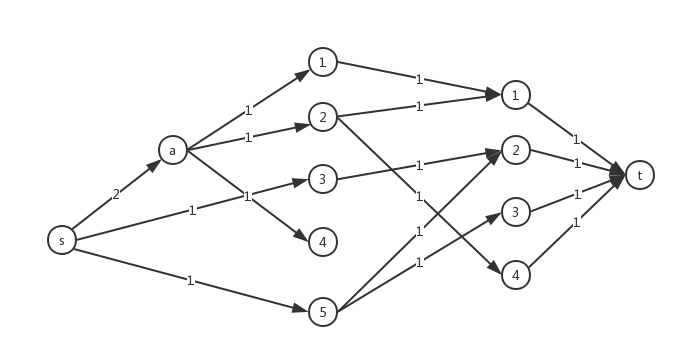# 输出方案

## 交叉分组#include
#include
#include
#include
#include
#include
#include
#include
#include
#include
#include
#include
#include

#define Re register
#define LL long long
#define U unsigned
#define FOR(i,a,b) for(Re int i = a;i <= b;++i)
#define ROF(i,a,b) for(Re int i = a;i >= b;--i)
#define SFOR(i,a,b,c) for(Re int i = a;i <= b;i+=c)
#define SROF(i,a,b,c) for(Re int i = a;i >= b;i-=c)
#define CLR(i,a) memset(i,a,sizeof(i))
#define BR printf("--------------------\n")
#define DEBUG(x) std::cerr << #x << '=' << x << std::endl

const int MAXN = 1000+5;
const int MAXM = 1000000+5;

struct Node{
struct Edge *first,*cur;
int level,num;
}node[MAXN];

struct Edge{
Node *s,*t;
Edge *next,*rev;
int cap,flow;
}pool[MAXM<<1],*frog = pool;

Edge *New(Node *s,Node *t,int cap){
Edge *ret = ++frog;
*ret = (Edge){s,t,s->first,NULL,cap,0};
return ret;
}

inline void add(int u,int v,int cap){
node[u].first = New(&node[u],&node[v],cap);
node[v].first = New(&node[v],&node[u],0);
node[u].first->rev = node[v].first;
node[v].first->rev = node[u].first;
}

int N,S,T;

bool bfs(Node *s,Node *t){
FOR(i,0,N){
node[i].cur = node[i].first;
node[i].level = 0;
}
std::queue q;q.push(s);
s->level = 1;
while(!q.empty()){
Node *v = q.front();q.pop();
for(Edge *e = v->first;e;e = e->next){
if(e->flow < e->cap && !e->t->level){
e->t->level = v->level + 1;
if(e->t == t) return true;
q.push(e->t);
}
}
}
return false;
}

int dfs(Node *v,Node *t,int limit=INT_MAX){
int flow;
if(v == t) return limit;
for(Edge *&e = v->cur;e;e = e->next){
if(e->flow < e->cap && e->t->level == v->level + 1)
if((flow = dfs(e->t,t,std::min(limit,e->cap-e->flow)))){
e->flow += flow;
e->rev->flow -= flow;
return flow;
}
}
return 0;
}

int dinic(){
int ret = 0,flow = 0;
while(bfs(&node[S],&node[T])){
while((flow = dfs(&node[S],&node[T]))) ret += flow;
}
return ret;
}

int n,m,sum;
int a[MAXN],b[MAXN];

int main(){
scanf("%d%d",&n,&m);
FOR(i,1,n) scanf("%d",a+i),sum += a[i];
FOR(i,1,m) scanf("%d",b+i);
S = 0,T = n+m+1;
N = T;
FOR(i,1,n) node[i].num = i;
FOR(i,1,m) node[i+n].num = i;
int ans=dinic();
//DEBUG(ans);DEBUG(sum);
if(ans != sum){
puts("0");return 0;
}
puts("1");
FOR(i,1,n){
for(Edge *e = node[i].first;e;e = e->next){
if(e->cap == e->flow)
printf("%d ",e->t->num);
}
puts("");
}
return 0;
}


# 拆点## 「BZOJ1738」

#include
#include
#include
#include
#include
#include
#include
#include
#include
#include
#include
#include
#include

#define Re register
#define LL long long
#define U unsigned
#define FOR(i,a,b) for(Re int i = a;i <= b;++i)
#define ROF(i,a,b) for(Re int i = a;i >= b;--i)
#define SFOR(i,a,b,c) for(Re int i = a;i <= b;i+=c)
#define SROF(i,a,b,c) for(Re int i = a;i >= b;i-=c)
#define CLR(i,a) memset(i,a,sizeof(i))
#define BR printf("--------------------\n")
#define DEBUG(x) std::cerr << #x << '=' << x << std::endl

const int MAXN = 2000 + 5;
const int MAXM = 500000 + 5;
#define int LL
struct Node{
struct Edge *first,*cur;
int level;
}node[MAXN];

struct Edge{
Node *s,*t;
Edge *next,*rev;
int cap,flow;
}pool[MAXM<<1],*frog = pool;

inline void init(){
CLR(pool,0);CLR(node,0);
frog = pool;
}

Edge *New(Node *s,Node *t,int cap){
Edge *ret = ++frog;
*ret = (Edge){s,t,s->first,NULL,cap,0};
return ret;
}

inline void add(int u,int v,int cap){
// printf("%lld %lld %lld\n",u,v,cap);
node[u].first = New(&node[u],&node[v],cap);
node[v].first = New(&node[v],&node[u],0);
node[u].first->rev = node[v].first;
node[v].first->rev = node[u].first;
}

int N,S,T;

bool bfs(Node *s,Node *t){
FOR(i,0,N){
node[i].cur = node[i].first;
node[i].level = 0;
}
std::queue q;
q.push(s);s->level = 1;
while(!q.empty()){
Node *v = q.front();q.pop();
for(Edge *e = v->first;e;e = e->next){
if(e->flow < e->cap && !e->t->level){
e->t->level = v->level + 1;
if(e->t == t) return true;
q.push(e->t);
}
}
}
return false;
}

int dfs(Node *v,Node *t,int limit=LLONG_MAX){
if(v == t) return limit;
int flow;
for(Edge *&e = v->cur;e;e = e->next){
if(e->flow < e->cap && e->t->level == v->level + 1){
if((flow = dfs(e->t,t,std::min(limit,e->cap - e->flow)))){
e->flow += flow;
e->rev->flow -= flow;
return flow;
}
}
}
return 0;
}

inline int dinic(){
int flow,ans = 0;
while(bfs(&node[S],&node[T])){
while((flow = dfs(&node[S],&node[T]))){
ans += flow;
}
}
return ans;
}

LL f[MAXN][MAXN];
int a[MAXN],b[MAXN];
int n,m,sum;

inline void Floyd(){
FOR(k,1,n){
FOR(i,1,n){
FOR(j,1,n){
f[i][j] = std::min(f[i][j],f[i][k] + f[k][j]);
}
}
}
}

struct Data{
int u,v,w;
}d[MAXM];

inline bool check(LL k){
init();
S = 0,T = 2*n+1;N = T;
FOR(i,1,n) FOR(j,1,n){
//if(i == j) continue;
}
int ans = dinic();//DEBUG(ans);
// BR;
return (sum <= ans);
}

int line[MAXM],top = 0;
const int INF = 1000000000ll*MAXN;
signed main(){
scanf("%lld%lld",&n,&m);
LL L = 0,R = 0;
FOR(i,1,n) FOR(j,1,n) f[i][j] = INF;
//DEBUG(INF);
FOR(i,1,n) f[i][i] = 0;
FOR(i,1,n) scanf("%lld%lld",a+i,b+i);
FOR(i,1,n) sum += a[i];
// FOR(i,1,n) FOR(j,1,n) printf("%lld%c",f[i][j],(j == n) ? '\n' : ' ');
FOR(i,1,m){
int u,v;LL w;
scanf("%lld%lld%lld",&u,&v,&w);
d[i] = (Data){u,v,w};
f[u][v] = f[v][u] = std::min(f[u][v],w);
R += w;
}
Floyd();
std::set S;
//FOR(i,1,n) FOR(j,1,n) printf("%lld%c",f[i][j],(j == n) ? '\n' : ' ');
FOR(i,1,n) FOR(j,1,n) if(f[i][j] != INF) S.insert(f[i][j]);
for(std::set::iterator it = S.begin();it != S.end();it++){
line[++top] = *it;
}
LL ans = -1;
L = 1,R = top;
//DEBUG(check(70));
while(L <= R){
LL mid = (L+R)>>1;
if(check(line[mid])) R = mid - 1,ans = mid;
else L = mid + 1;
}
printf("%lld\n",ans == -1 ? ans : line[ans]);
return 0;
}


# 增加限制点Last modification：April 7th, 2020 at 10:14 pm